# Number Cardinal number Ordinal number Fraction Decimal House

• Slides: 13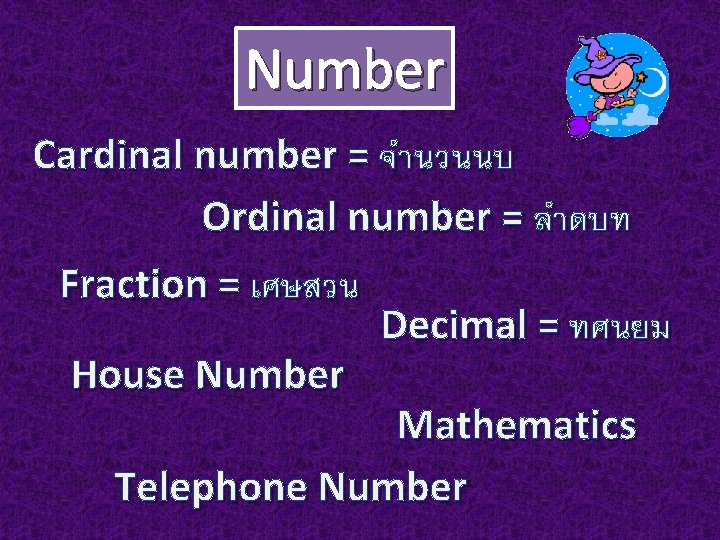Number Cardinal number = จำนวนนบ Ordinal number = ลำดบท Fraction = เศษสวน Decimal = ทศนยม House Number Mathematics Telephone Number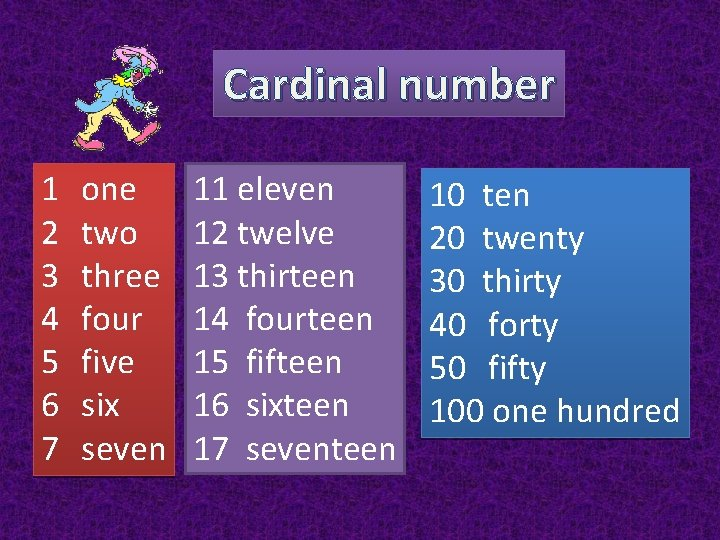Cardinal number 1 2 3 4 5 6 7 one two three four five six seven 11 eleven 12 twelve 13 thirteen 14 fourteen 15 fifteen 16 sixteen 17 seventeen 10 ten 20 twenty 30 thirty 40 forty 50 fifty 100 one hundredCardinal number 101 one hundred and one 1, 000 one thousand 10, 000 ten thousand 100, 000 one hundred thousand 1, 000 one millionOrdinal number 1 st first 2 nd second 3 rd third 4 th fourth 5 th fifth 6 th sixth 7 th seventh 11 th 12 th 13 th 14 th 15 th 16 th 20 th eleventh twelfth thirteenth fourteenth fifteenth sixteenth twentieth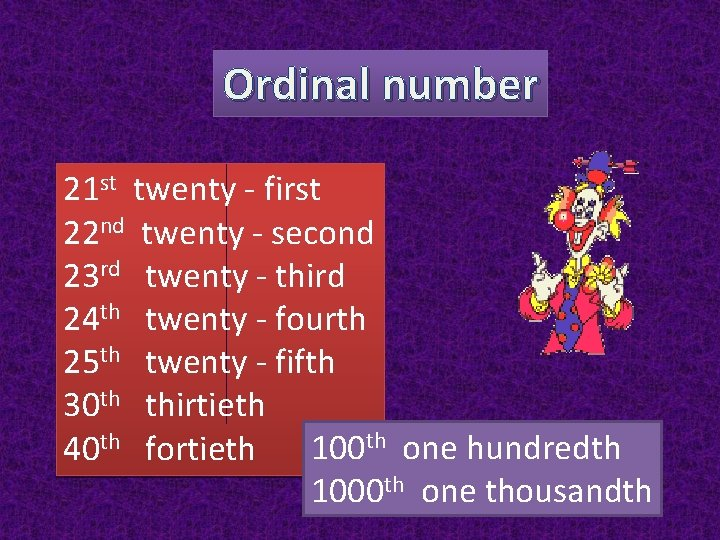Ordinal number 21 st twenty - first 22 nd twenty - second 23 rd twenty - third 24 th twenty - fourth 25 th twenty - fifth 30 th thirtieth 100 th one hundredth 40 th fortieth 1000 th one thousandth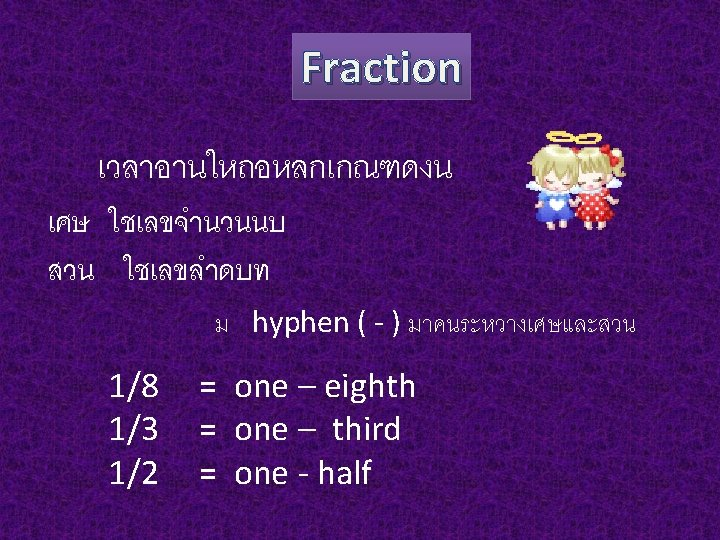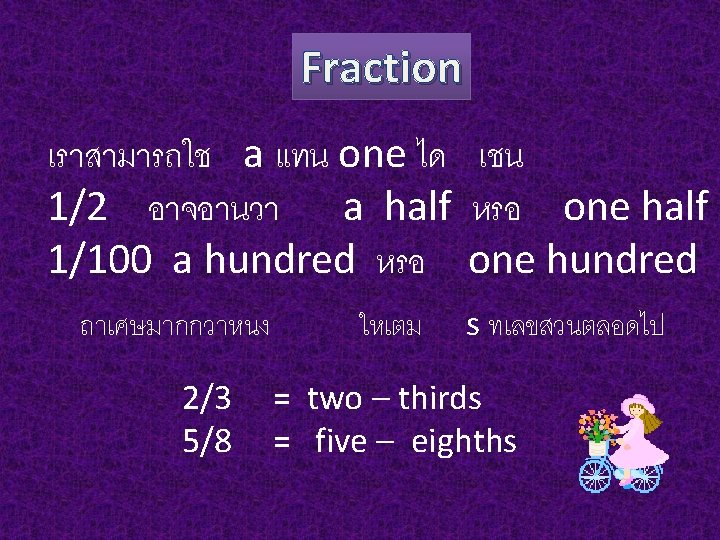Fraction ถาเศษมตวเลขตงแตสองตวขนไปหรอตงแตหลกสบขนไป ใช over แทน hyphen ( - ) ระหวางเศษและสวน 32/51 = thirty-two over fifty-first 749/4000 = seven hundred and forty-nine over four thousandths 2/3 5/8 = two – thirds = five – eighths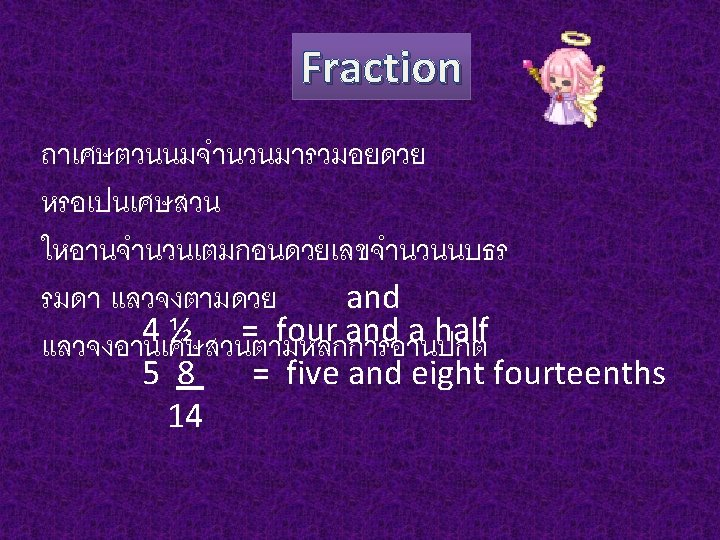Decimal เลขหนาจดทศนยม อานแบบเลขจำนวนนบธรรมดา จด อานวา point เลขหลงจดอานทละตว 1, 547. 035 อานวา one thousand, five hundred and forty-seven point oh three five 0. 125 = zero point one two five 0. 33 = zero point three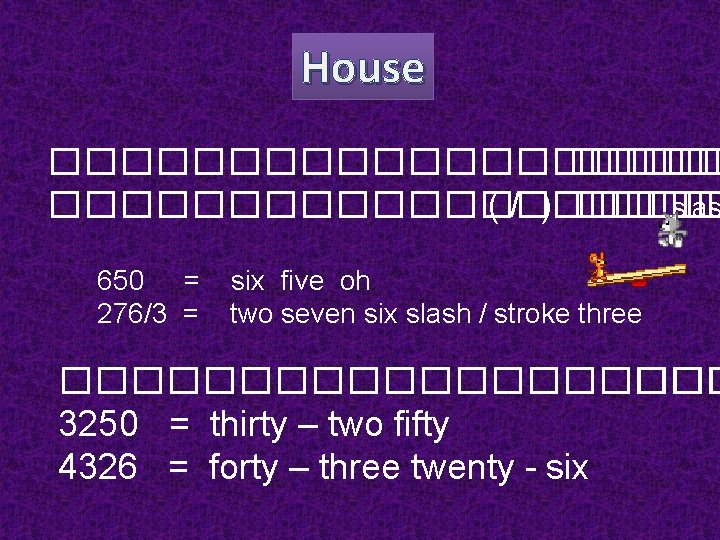House ������������ ( / ) ����� slas 650 = 276/3 = six five oh two seven six slash / stroke three ���������� ��� 3250 = thirty – two fifty 4326 = forty – three twenty - sixTelephone Number Tel. 037 -321430 oh three seven three two one four three oh Tel. 02 -546 -2500 oh two five four six two five hundred (five double oh )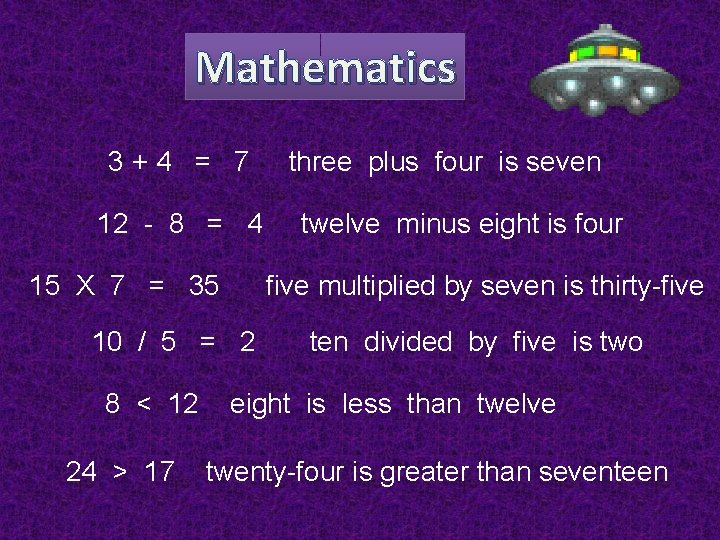Mathematics 3+4 = 7 12 - 8 = 4 15 X 7 = 35 24 > 17 twelve minus eight is four five multiplied by seven is thirty-five 10 / 5 = 2 8 < 12 three plus four is seven ten divided by five is two eight is less than twelve twenty-four is greater than seventeen